Get instant live expert help with Excel or Google Sheets“My Excelchat expert helped me in less than 20 minutes, saving me what would have been 5 hours of work!”

#### Post your problem and you’ll get Expert help in seconds.

Your message must be at least 40 characters
Our professional Expert are available now. Your privacy is guaranteed.

# Series of dates by year

Excel has made working with dates simpler than ever before. We can generate a series of dates by year using the DATE function. The DATE function takes the YEAR, MONTH and DAY arguments. In this tutorial, we will learn how to generate a series of dates by year.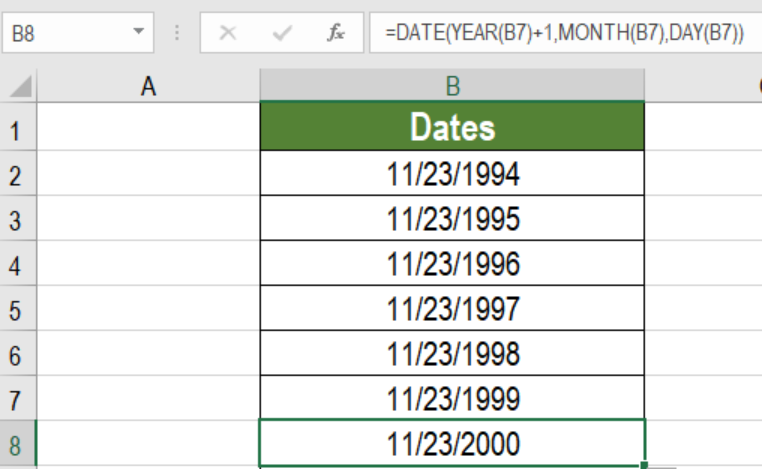Figure 1: Example of How to Generate a Series of Dates by Year

## Generic Syntax

`=DATE(YEAR(date)+1,MONTH(date),DAY(date))`

## How this Formula Works

In order for this formula to work, we first need a hard coded date. The Date function takes this date and extracts the year, month, and day values. YEAR (DATE) + 1 adds 1 to the year. With this information, the DATE function generates a new date with the same month and day but the next year. Thus, this formula generates a series of new dates by year.

## Setting Up Data

The following example contains a hard coded date in cell B1.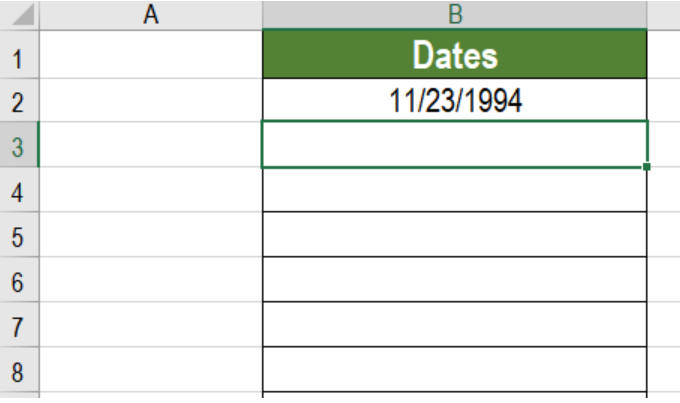Figure 2: The Hard-Coded Date to Work With

To generate series of dates by year in column B

• Go to cell B3.
• Click Formulas>Insert Function. In the window that pops up, type DATE in the search box and click Go.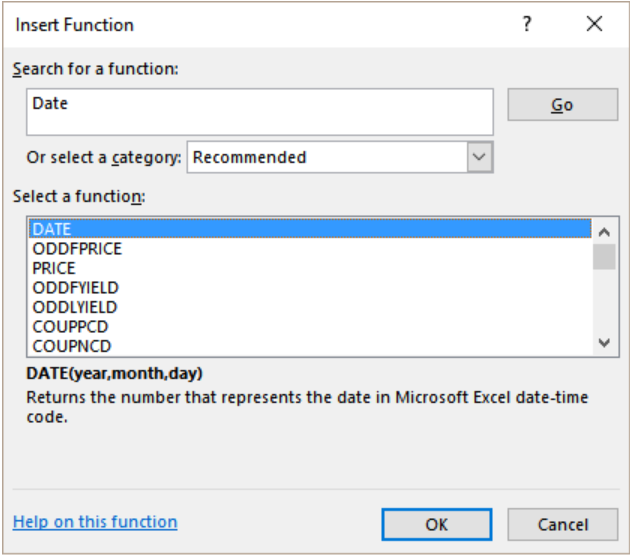Figure 3: Searching the DATE Function

• This will open a pop up named Select a Function. Select the function DATE and press Ok.
• Next, we need to provide the inputs for the YEAR, MONTH and DAY values. In this case, the values will be `YEAR(B2)+1, MONTH(B2) and DAY(B2)`.
• Now, we need to click OK. This will enter the formula `=DATE (YEAR(B2)+1,MONTH(B2),DAY(B2)) `to cell B3.
• Finally, we will need to drag the formula from cells B3 to B8 to generate series of dates by year.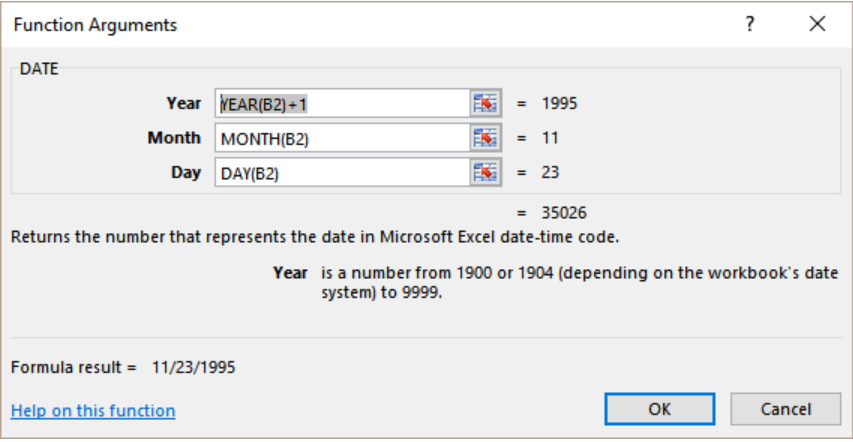Figure 4: Providing the Arguments

• Press Ok.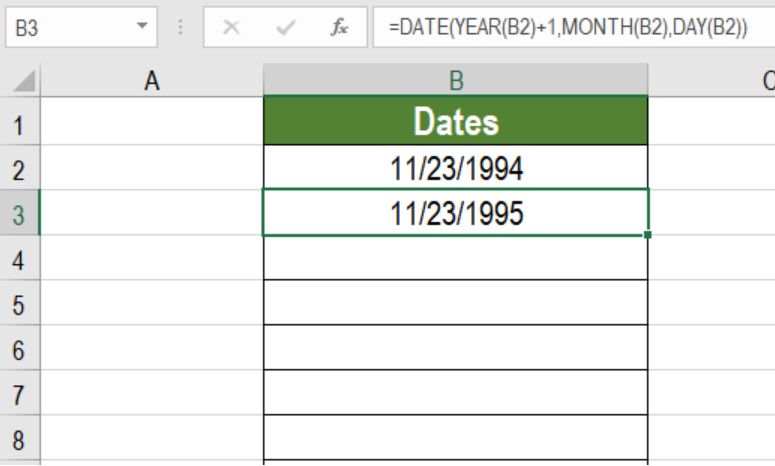Figure 5: Completing the formula

Column B will now have a series of dates by year following the date in B2.

The DATE function is very handy working with dates. Here, with the help of this function we can generate a series of dates by year. By changing the MONTH and DAY parameters, we can also generate different dates.

Most of the time, the problem you will need to solve will be more complex than a simple application of a formula or function. If you want to save hours of research and frustration, try our live Excelchat service! Our Excel Experts are available 24/7 to answer any Excel question you may have. We guarantee a connection within 30 seconds and a customized solution within 20 minutes.

### Did this post not answer your question? Get a solution from connecting with the expert.Another blog reader asked this question today on Excelchat:
Solution examplesa date formula that results in showing the date as 29/12/00 (date format as dd/mm/yy) - use column BA to calculate 364 days (same date format) in column BS, if BA blank, then calculate 364 days using column AX. Results for column BA are correct. However, if column BA is empty, then it should calculate using column AX, which has data, but the result is always 29/12/00, regardless of the date in column AX. I have used this formula with success in another workbook, but this file wont work! Formula: =IF(AND(LEN(\$BA74)=0,LEN(\$AX74)=0),"",IF(LEN(\$BA74)=0,DATE(YEAR(\$AX74),MONTH(\$AX74),DAY(\$AX74)+364),DATE(YEAR(\$BA74),MONTH(\$BA74),DAY(\$BA74)+364)))
Solved by A. L. in 60 minsHOW CAN I COUNT NUMBERS PER DAY FOR A MONTH AS TOTAL?
Solved by F. F. in 16 minshow do I derive the monthly sales from a date and revenue column
Solved by A. U. in 23 minsI have multiple columns on my spread sheet and want to switch from A-Z to Z-A based on values in column A
Solved by V. H. in 38 minsConcat formula is incorrectly formatting the date in column C. Currently column B has dd/mm/yyyy and I require mm/dd/yyyy
Solved by Z. Y. in 31 mins## Subscribe to Excelchat.coAnother blog reader asked this question today on Excelchat: